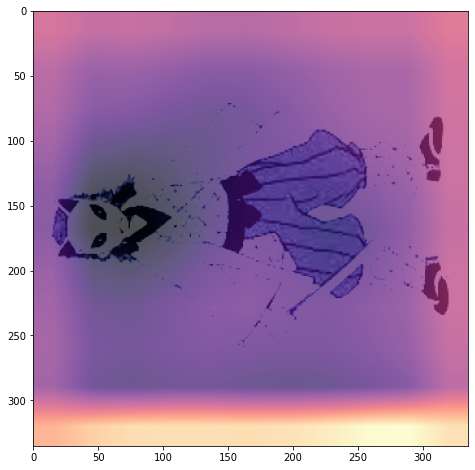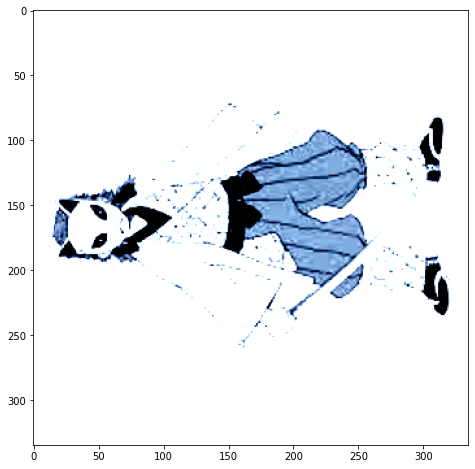# Visualising model using hooks returns unexpected results

I am a pytorch beginner and tried to visualize the last convolution layer of the resnet using feed forward hooks. I tried to create a heatmap that shows what part of the image ,according to the resnet model, contained the most important features. However, the resulting heatmap is not at all what you might expect. I’m not sure if the code I have written is wrong, or is there something I don’t understand about the hidden layers or the hooks in pytorch.

``````img, label = next(iter(valid_dl))
img = img.to(device)

weights = []
def get_layer(module, input, output):
weights.append(output)

model.layer4.conv1.register_forward_hook(get_layer)
out = model(img)
activated = weights
mean_act = activated.mean(0)
``````

The code for the heatmap is as follows:

``````fig , ax = plt.subplots()
ax.imshow(img.cpu().permute(2, 1, 0))
ax.imshow(mean_act.cpu().detach(), alpha = 0.5, extent=(0, 335, 335, 0),
interpolation = 'bilinear', cmap = 'magma' )
``````

But the resulting Image is unclear:Here is the original image without the heatmap:I first hooked out the last convolutional layer of an arbitrary image in the restnet, and then took the mean over the 512 channels and ended up with a tensor of size 11 by 11. From what I understand this tensor should contain information about how the model classified our image. The heat map above shows that the bottom part of the image contributed the most to the classification. As I mentioned I’m a beginner and I am not sure that if my reasoning is correct. I would appreciate some insight on this subject.